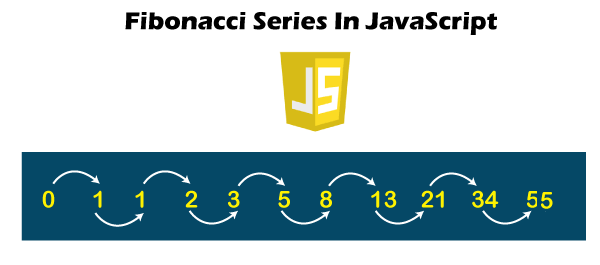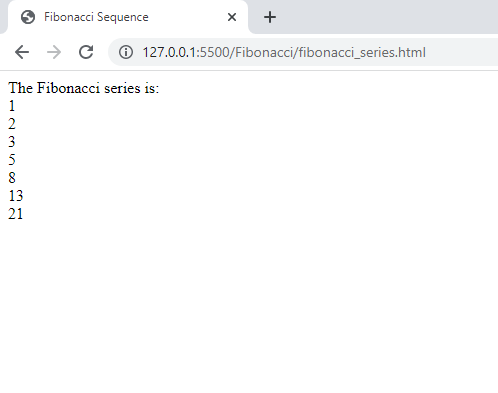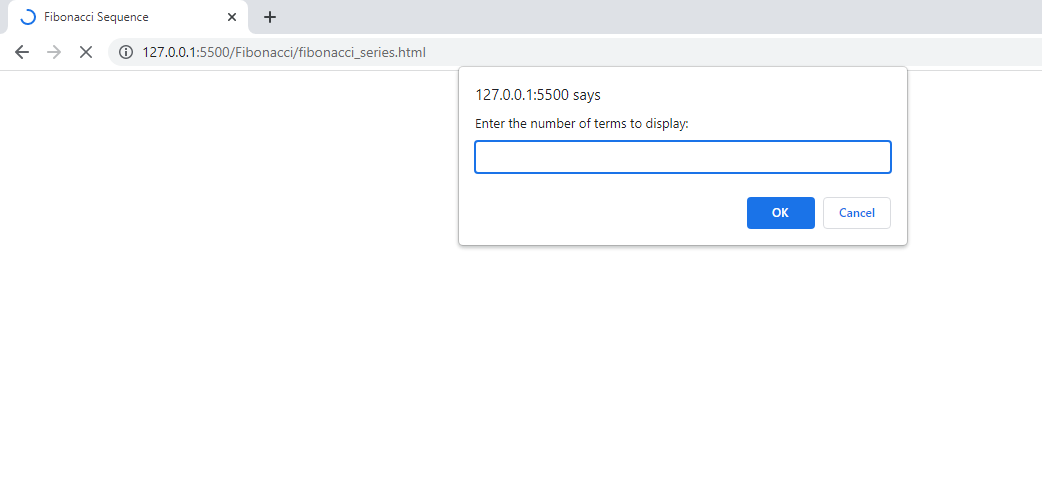# JavaScript Program For Fibonacci Series

in STEMGeeks2 years agoImage Source

Here in this post, we will be writing a JavaScript code that helps to find the fibonacci sequence upto the number of terms that user has specified. We will just need a for-loop concept. Also, I preferred solving it through functional approach: creating a function. For anyone who don't know about Fibonacci sequence: it is a sequence in which a current item is the sum of its preceeding two items. The following equation helps to find the Fibonacci sequence in mathematics.

### Xn = X(n-1) + X(n-2) for n >=3.

``````<!DOCTYPE html>
<html>
<title>Fibonacci Sequence</title>
<script>

function findFibonacci(x,y)
{
first_term = x;
second_term = y;
number_of_terms = 7;
document.write("The Fibonacci series is: ")
for (var i=1; i<=number_of_terms; i++)
{
document.write("<br>" + x);
next_term = x+y;
x=y;
y=next_term;
}
}
findFibonacci(1,2);
</script>
<body>
</body>
</html>
``````

In the above code, we specified the first term of sequence to be 1 and the second term to be 2. We want to display the sequence upto seventh terms and we get the output as below:For now, we haven't prompted user to specify their own first term and second term value and also the number of terms that they want to display. It is good to do this by using DOM manipulation which I haven't started yet. However you can also do this by using `prompt()` function that pop-ups a dialog box in your browser window asking user for the input.

For example: in the above code, instead of `number_of_terms = 7;`, we can replace it with:

`var number_of_terms = prompt("Enter the number of terms to display:");`

When you run the output, you will get the same as following and you can enter your desired value in that textbox and the program will show your desired output.